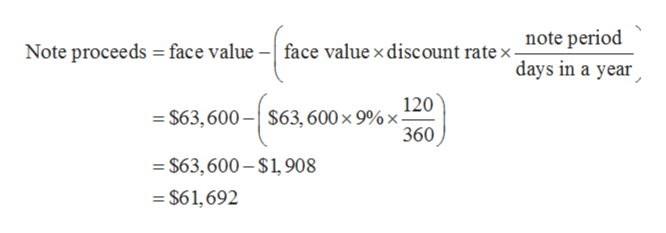# Proceeds from Notes PayableOn May 15, Maynard Co. borrowed cash from Texas Bank by issuing a 120-day note with a face amount of \$63,600. Assume a 360-day year.Required:a. Determine the proceeds of the note, assuming the note carries an interest rate of 9%.\$b. Determine the proceeds of the note, assuming the note is discounted at 9%.\$

Question
485 views

Proceeds from Notes Payable
On May 15, Maynard Co. borrowed cash from Texas Bank by issuing a 120-day note with a face amount of \$63,600. Assume a 360-day year.
Required:
a. Determine the proceeds of the note, assuming the note carries an interest rate of 9%.
\$
b. Determine the proceeds of the note, assuming the note is discounted at 9%.
\$

check_circle

Step 1

Notes payable is a liability account. It records the face value of the promissory notes that a company issues. It shows the amount that a company must pay to others.

Step 2

Given:

Face value of note: \$63,600

Note is issued for 120 days.

Interest rate: 9%

Part (a)

Calculation of note proceed at interest rate of 9% is:

Note proceeds at interest rate of 9% will be equal to the face value of the note issued. So, note proceeds is \$63,600.

Step 3

Face value of note : \$63,600

Discount rate : 9%

Note period is 120 days.

Days in a year is 3...help_outlineImage Transcriptionclosenote period Note proceeds face value -face value x discount rate x- days in a year 120 = \$63,600-\$63, 600 x 9% x- 360 \$63,600-\$1,908 \$61,692 fullscreen

### Want to see the full answer?

See Solution

#### Want to see this answer and more?

Solutions are written by subject experts who are available 24/7. Questions are typically answered within 1 hour.*

See Solution
*Response times may vary by subject and question.
Tagged in